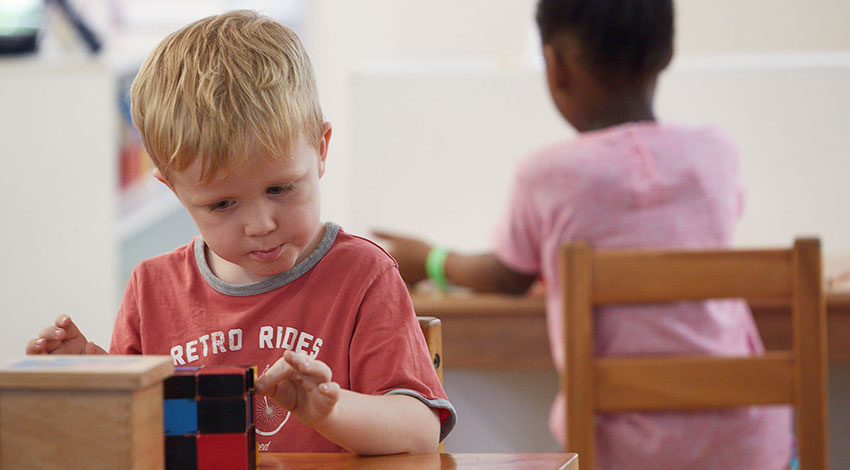1 April 2020 - 12:00am

The study of mathematics is a reflection of the human tendencies for investigation and orientation, for order and classification, for reasoning and making judgements, and for calculating and measuring. In the Montessori Children’s House (children 3-6 years of age), when mathematical concepts are first presented to children, they are embodied in concrete materials.

Mathematics in the Children’s House builds on and extends the exercises of practical life and the exercises of the senses, as well as the many mathematical experiences children encounter incidentally in their daily lives, including experiences with:

• visual representation of mathematical concepts
• pattern and order
• problem solving
• cardinal and ordinal numbers
• place value
• operations (addition, subtraction, multiplication, division)
• fractions
• spatial relations e.g. placement of objects, spatial patterns, one-to-one correspondence of objects and two-dimensional shapes
• measurement e.g. length, mass, time, temperature, volume, perimeter, area
• word problems (addition, subtraction, multiplication, division)

As they work with the exercises of the senses, children are making judgements in relation to distance, dimension, graduation, identity, similarity and sequence. Building on this foundation, the Montessori mathematics materials introduce children to:

• counting (from 1 to 10, 10 to 90, linear 1 to 100 and 1000, and skip-counting as an introduction to multiplication)
• place value to four digits
• number operations (addition, subtraction, multiplication, division).

The base ten number system is represented for children in concrete form using golden beads organised so they vary simultaneously in quantity, size, mass and geometric shape. Children are also given the corresponding symbol for each quantity. In this way, children experience relations between the hierarchies of the system in multiple ways. Using this material in active and enjoyable games, children learn to add, subtract, multiply and divide. This material supports the heightened sensitivity for number children tend to experience around the age of four.

The progression of the Montessori mathematics curriculum in the Children’s House follows a five-step sequence.

1. Introduction of Concrete Materials: (The quantity is presented in isolation.)

Concepts are presented in a concrete form children can manipulate. Children are given accurate language to talk about the concrete impressions. Only after they have experienced the concrete material are they given the symbolic mathematical notation.

2. Introduction of Symbols: (The visually recognised symbols are offered in isolation.)

When the child is comfortable with the concrete representation and the oral language, mathematical symbols are introduced.

3. Association of the Concrete with the Symbols: (Accurate language is the link.)

Only after the child has completed the first two steps are the concrete materials and symbols combined.

4. Practice

After being presented with new information children need the opportunity for repetition. Children are offered a way to practise using and remembering this new knowledge and integrating it with what is already known. They have the opportunity to build and consolidate the knowledge through use of the materials, until it becomes automatic.

5. Self Assessment

Children are given exercises to affirm or verify their own understanding and knowledge, and to establish whether they are ready for the next exercise.

Children work through the Montessori mathematics exercises and games in a supported and incremental way. There is no pressure for children to move onto a new topic before mastering the one they are working on. Students are free to progress through the mathematics curriculum following their own interests, so not all children complete the activities in the same sequence or in the same timeframe.